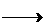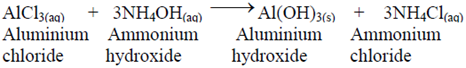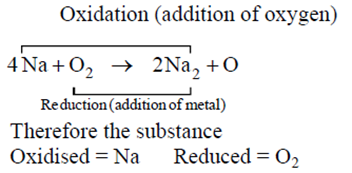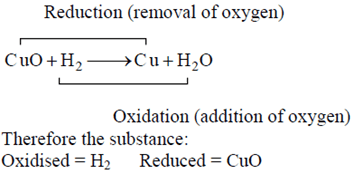# CBSE 10th Chemistry | Chemical Reactions -NCERT Questions with Answers

## Chemical Reactions -NCERT Questions with Answers

### Question 1. Why should a magnesium ribbon be cleaned before burning in air?

The magnesium ribbon which we use has a coating of “basic magnesium carbonate” on its surface, which is formed by a slow action of moist air on it. Therefore before burning it in air, it is cleaned by a sand paper which remove the protective layer of basic magnesium carbonate from the surface of magnesium ribbon, so that it can easily burn.

### Question 2. Write the balanced equation for the following chemical reactions

#### (iii) Sodium + WaterSodium hydroxide + Hydrogen

(ii) 3BaCl2(s) +Al 2(S04)3(aq)3BaSO4{s) + 2AlCl3(aq)

(iii) 2Na(s) + 2H2O(l)2NaOH(aq) + H2(g)

### Question 3. Write a balanced chemical equation with state symbols for the following reactions.

#### (ii) Sodium hydroxide solution (in water) reacts with hydrochloric acid solution (in water) to produce sodium chloride solution and water.

(i) BaCl2(aq) + Na2SO4(aq)BaSO4(s) + 2NaCl(aq)

(ii) NaOH(aq) + HCl(aq)NaCl(aq) + H2O(aq)

### Question 4. A solution of a substance ‘X’ is used for white washing.

#### (ii) Write the reaction of the substance ‘X’ named in (i) above with water,

(i) Quick lime-CaO

### Question 5. Why is the amount of gas collected in one of the test tube in activity 1.7 (Refer NCERT) double of the amount collected in the other? Name this gas.

The gases formed at two electrodes are produced due to decomposition of water on passing electricity. Since the electrolysis of water produces 2 volume of hydrogen gas and 1 volume of oxygen gas, thus we can say that the ratio of hydrogen and oxygen in water is 2:1 by volume and hence the amount of gas collected in one test tube will be double of the amount collected in other.

### Question 6. Why does the colour of copper sulphate solution changes when an iron nail is dipped in it?

As iron is more reactive than copper thus it displaces copper from copper sulphate solution.

(Blue)                 (Green)

In this way concentration of copper sulphate decreases while concentration of ferrous sulphate increases. As a result colour changes from blue to green.

### Question 7. Give an example of a double displacement reaction other than the one given in Activity 1.10 (Refer NCERT).### Question 8. Identify the substances that are oxidised and the substances that are reduced in the following reactions.

#### (ii) CuO(s) + H2(g)Cu(s) + H2O(l)

Ans. (i)